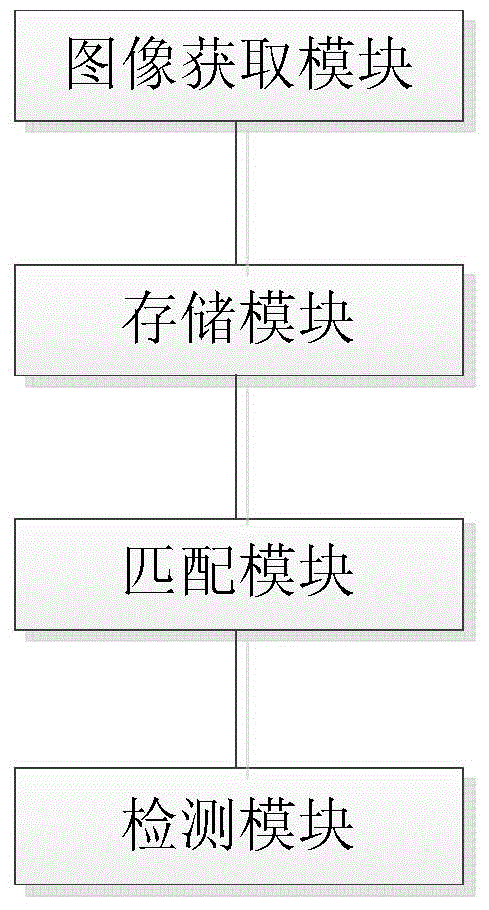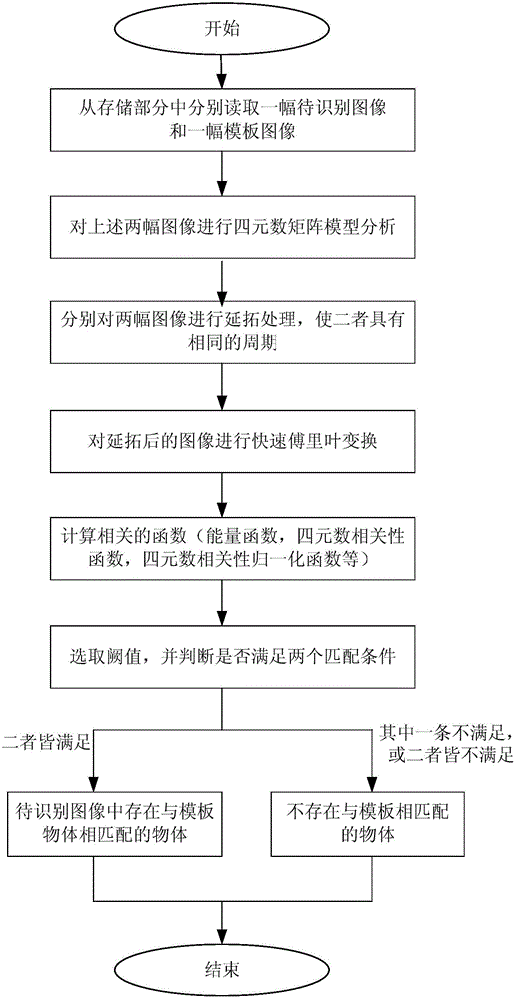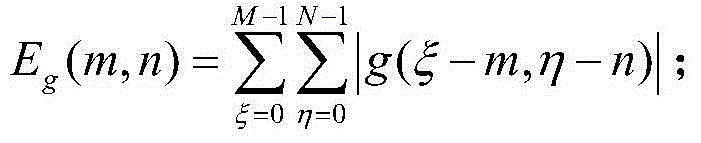# RGB vector matching rapid-recognition system and method

## A vector matching and recognition system technology, applied in character and pattern recognition, image data processing, instruments, etc., can solve the problems of destroying the correlation of color images, increasing the amount of calculation, and affecting the accuracy and robustness of matching algorithms.

Active Publication Date: 2014-02-05
SOUTH CHINA UNIV OF TECH
4 Cites 18 Cited by

## AI-Extracted Technical Summary

### Problems solved by technology

The traditional color image matching method divides the image into three components (RGB), regards the image of each component as a grayscale image, performs matching processing in each component, and then synthesizes the final image according to the weight value of each color However, this c...
View more

## Abstract

The invention discloses an RGB vector matching rapid-recognition system. The RGB vector matching rapid-recognition system comprises an image obtaining module, a storage module, a matching module and a detection module, wherein the image obtaining module is used for obtaining an image to be recognized, the storage module is used for storing a template image and the obtained image to be recognized, the matching module is used for moving the template image, searching for a unit which is matched with the template image in the image to be recognized, and using the quaternion correlation to judge whether an object which is the same as a template object in shape, size, color and brightness exists, and the detection module judges the degree of matching between the image to be recognized and the template image according to the calculation result of the matching module, and detects whether a PCB has the welding spot defect or not. The RGB vector matching rapid-recognition system and method are small in amount of calculation, the relevance between colors cannot be destroyed, and accuracy is high.

Application Domain

Image analysisCharacter and pattern recognition

Technology Topic

Brightness perceptionQuaternion +1

## Image

•••## Examples

• Experimental program(1)

### Example Embodiment

 Such as figure 1 As shown, an RGB vector matching rapid recognition system includes an image acquisition module, a storage module, a matching module, and a detection module.
 The image acquisition module is a single-lens color light source stereo vision system used to acquire the image to be recognized, wherein the single-lens color light source stereo vision system is a single-lens three-ring special color light source structure, and chromaticity information is added;
 The storage module is used to store the template image and the acquired image to be recognized;
 The matching module is used to move the template image, search for the unit matching the template image in the image to be recognized, and use the quaternion correlation to determine whether there is an object with the same shape, size, color and brightness as the template object: first use The quaternion matrix model describes the image to be recognized, that is, the color value of each position in the image to be recognized can be represented by a quaternion vector; then the template image is represented by a quaternion f(ξ,η), and a quaternion is used g(ξ,η) represents the image to be recognized on the PCB, f(ξ,η) and g(ξ,η) are respectively M×N, P×Q; finally calculate whether there is a certain position in the image to be recognized (m 0 ,n 0 ) Such that g(m,n)=f(m-m 0 ,n-n 0 );
 Detection module, the detection module judges the matching degree between the image to be recognized and the template image according to the calculation result of the matching module. If the image to be recognized exists in a certain position (m 0 ,n 0 ) Such that g(m,n)=f(m-m 0 ,n-n 0 ), it means that the position (m s ,n s ) There is an object that matches the template image, and the color image recognition is completed.
 A fast recognition method for RGB vector matching, such as figure 2 As shown, the steps in the following sequence are included:
 First, store the color image of the PCB board to be identified collected by the single-lens color light source stereo vision system in the image acquisition module into the storage module;
 Next, the matching module reads a to-be-recognized image and a template image in the storage module, and establishes a quaternion matrix model of the above two images; let quaternion q=q r +q i i+q j j+q k scalar part q in k r =0, get the chromaticity value of the RGB three-color space color image represented by quaternion, written as f(x,y)=R(x,y)i+G(x,y)j+B(x,y) )k, where R(x,y), G(x,y), B(x,y) respectively represent the gradation values ​​of the RGB three-color space of the image at the position (x,y);
 Furthermore, it is known from the convolution theorem that the Fourier transform of function convolution is the product of the function Fourier transform, so the two can be converted to each other through operations; but when the convolution function is obtained from the Fourier transform, the period of the function is There are certain constraints on the performance, otherwise it will lead to wrong conclusions; therefore, to ensure that the template image function f(ξ,η) and the image function to be recognized g(ξ,η) have the same period C in the x direction and the same in the y direction The period D must be extended; periods C and D are both exponential powers of 2 and satisfy C ≥ M + P - 1 D ≥ N + Q - 1 , Extend the template image f(ξ,η) and the image to be recognized g(ξ,η) to get f e (ξ,η) and g e (ξ,η), so that the two have the same period;
 The traditional correlation technology applied to gray-scale images, the normalized form of the correlation function is:
 R ( x , y ) = X i = 0 M - 1 X j = 0 N - 1 f ( i , j ) h ( i + x , j + y ) X i = 0 M - 1 X j = 0 N - 1 | f ( i , j ) | 2 X i = 0 M - 1 X j = 0 N - 1 | h ( i + x , j + y ) | 2
 As the brightness of the image changes, R(x,y) also changes. If and only if the template image and the image at the corresponding position of the image to be recognized completely match, R(x,y)=1; similar to the traditional correlation The normalized form of the quaternion correlation function suitable for color images is obtained as follows:
 h ( m , n ) = b ( m , n ) E f E h ( m , n )
 among them,
 b(m,n) is the quaternion correlation function, expressed as b(m,n)
 E f Is the energy function of the template image, expressed as
 E g (m,n) is the energy function of the image to be recognized, expressed as Among them, 0≤m≤P-M+1,0≤n≤Q-N+1;
 In the quaternion correlation calculation process, in view of the high complexity of the time-domain discrete convolution calculation, the time-consuming is too long, the real-time requirements in practical applications cannot be well met; therefore, the present invention combines the time-domain The convolution operation is converted to a simple Fourier function product operation in the frequency domain, and it is applied to the calculation of the quaternion correlation, thereby improving the calculation speed; the Fourier transform of the quaternion correlation is obtained by the following formula achieve:
 h ( x , y ) = IQFT ( F ( q 3 ) ( w , v ) G a ( q 3 ) ( w , v ) ‾ - F ( q 3 ) ( - w , - v ) · j H b ( q 3 ) ( - w , - v ) ) ,
 Where IQFT is the inverse quaternion Fourier transform:
 f ( x , y ) = ( 4 π 2 ) - 1 ∫ - ∞ ∞ ∫ - ∞ ∞ F ( q 3 ) ( w , v ) · e μ 1 ( wx + vy ) dwdv
 Further, select the threshold P 1 , P 2 , D 1 , So that it meets the condition P 1 <1 2 , D 1 <1, and judge |g(m s ,n s )| Is the value in the interval (P 1 ,P 2 ] And satisfy the following formula:
 ρ = | g r ( m s , n s ) | | g r ( m s , n s ) | + | g i ( m s , n s ) | + | g j ( m s , n s ) | + | g k ( m s , n s ) | d 1
 Finally, the detection module determines whether the above conditions are met: if the above conditions are all met, it means that the position (m) in the image g(ξ,η) to be recognized is s ,n s ) There is an object with the same shape, size, color and brightness as the template object. If one of the conditions is not met, or both are not met, there is no object matching the template; so far, the PCB board color image Recognition is complete.
 The above-mentioned embodiments are preferred embodiments of the present invention, but the embodiments of the present invention are not limited by the above-mentioned embodiments, and any other changes, modifications, substitutions, combinations, etc. made without departing from the spirit and principle of the present invention Simplified, all should be equivalent replacement methods, and they are all included in the protection scope of the present invention.

## PUM## Description & Claims & Application Information

We can also present the details of the Description, Claims and Application information to help users get a comprehensive understanding of the technical details of the patent, such as background art, summary of invention, brief description of drawings, description of embodiments, and other original content. On the other hand, users can also determine the specific scope of protection of the technology through the list of claims; as well as understand the changes in the life cycle of the technology with the presentation of the patent timeline. Login to view more.
Who we serve
• R&D Engineer
• R&D Manager
• IP Professional
Why Eureka